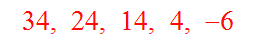# 9118 Arithmetic Any Term From First 3

3 Questions | Total Attempts: 56Settings• 1.
From the arithmetic sequence below, find the 19th Term.
• 2.
From the arithmetic sequence below, find the 36th Term.
• 3.
From the arithmetic sequence below, find the 18th Term.
• 4.
From the arithmetic sequence below, find the 18th Term.
• 5.
From the arithmetic sequence below, find the 37th Term.
• 6.
From the arithmetic sequence below, find the 18th Term.
• 7.
From the arithmetic sequence below, find the 14th Term.
• 8.
From the arithmetic sequence below, find the 30th Term.
• 9.
From the arithmetic sequence below, find the 18th Term.
• 10.
From the arithmetic sequence below, find the 35th Term.
Related TopicsBack to top